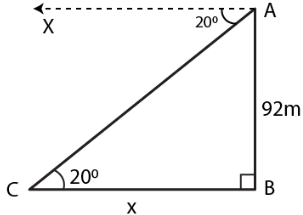Guru

# . From the top of a cliff 92 m high, the angle of depression of a buoy is 200. Calculate to the nearest metre, the distance of the buoy from the foot of the cliff.

• 0

sir this is the question from the book -ML aggarwal( avichal publication) class 10th , chapter20 , heights and distances
we have been given that  From the top of a cliff 92 m high,
the angle of depression of a buoy is 200. we

have to Calculate to the nearest metre, the distance of the buoy from the foot of the cliff.

question no 7 , heights and distances , ICSE board, ML aggarwal

Share

1. Consider AB as the cliff whose height is 92 m

C is buoy making depression angle of 200We know that

∠ACB = 200

Take CB = x m

In a right angle triangle ABC

cot θ = BC/AB

Substituting the values

cot 200 = x/92

By cross multiplication

x = 92 × cot 200

So we get

x = 92 × 2.7475

x = 252.7700 m

Hence, the distance of the buoy from the foot of the cliff is 252.77 m.

• 0